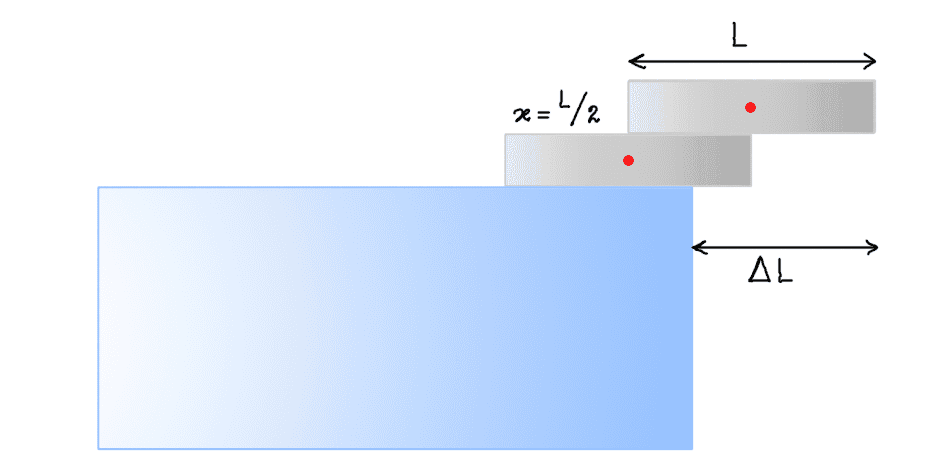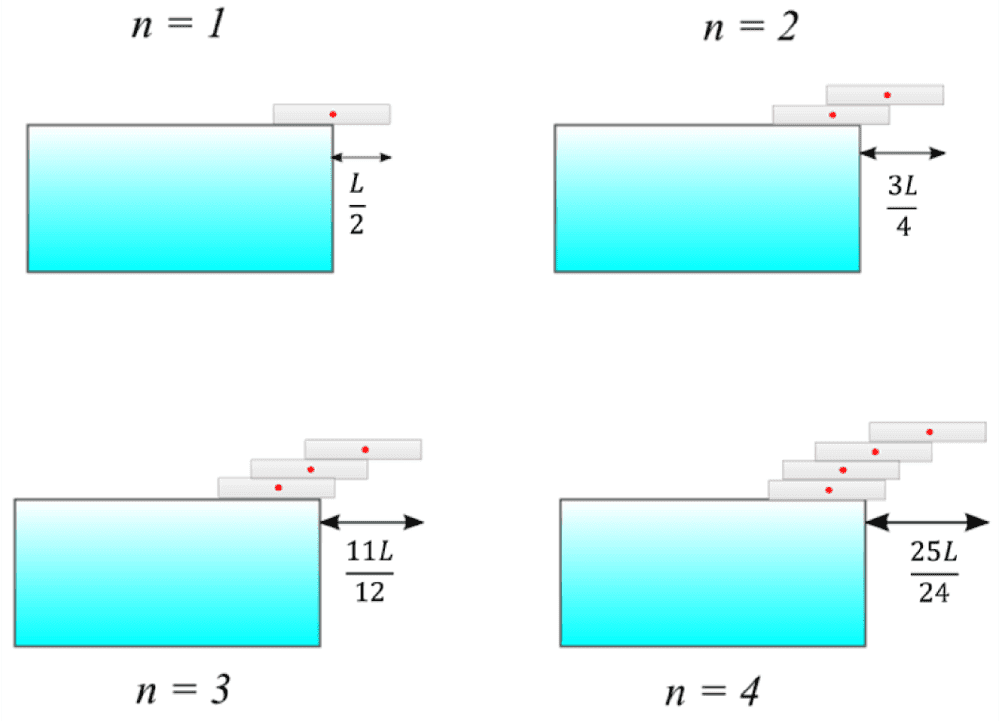# Center of gravity and over-hanging blocks

brotherbobby
Homework Statement:
Two identical blocks are stacked from the edge of a table, as the figure below shows. If each block has a length ##L##, what is the maximum overhang possible?
Relevant Equations:
The center of mass (or gravity) : ##x_{\text{CM}} = \frac{\Sigma m_i x_i}{\Sigma m_i}##The red dots show the CM of each block. ##x## is the amount by which the upper block overhangs the lower block. The blue (dashed) line shows the CM of the combination. For maximum overhanging, this line lies on the edge of the table below. By symmetry, the CM lies exactly midway between the two CMs of the individual blocks - hence each CM must be a distance ##\frac{x}{2}## from the CM of the combination (on either side). The amount of overhanging ##\Delta L = \frac{L+x}{2}##. Question is then, how to relate ##x## to ##L##?

I could not progress further.

Staff Emeritus
Homework Helper
Gold Member
You have correctly identified when both blocks together will fall off if they were glued together, but they are not. What happens as you increase ##x##? What will happen to the upper and lower blocks, respectively?

brotherbobby
You have correctly identified when both blocks together will fall off if they were glued together, but they are not. What happens as you increase ##x##? What will happen to the upper and lower blocks, respectively?

As you increase ##x## the upper block moves to the right, relative to the lower. The CM of the combination also moves to the right. Now yes, if the blocks were glued together, the combination would fall over.

But they are not. So as ##x## increases, the upper block falls while the lower block remains where it is.

Staff Emeritus
Homework Helper
Gold Member
So at what ##x## does the upper block fall over?

brotherbobby
So at what ##x## does the upper block fall over?
It seems that the upper block would remain on the lower block for as long as its CM remains between the left and right extremes of the lower block.
Hence, when ##x\geq \frac{L}{2}##, the upper block falls over ##-## just maintaining its position at the very right when ##x=\frac{L}{2}##.

Staff Emeritus
Homework Helper
Gold Member
So in the end, the maximal ##\Delta L## is given by?

brotherbobby
I must redraw to be able to visualise the thought development which your queries provoke. My original diagram up there is clearly inaccurate (given the distances I now realize).Ok so with a better drawing, I find that, given ##x = \frac{L}{2}\Rightarrow \Delta L = \frac{L+\frac{L}{2}}{2} \Rightarrow \boxed{\Delta L = \frac{3L}{4}}##, using the formula I derived above.

Again, from the redrawn figure above, we see that ##\boxed{\Delta L} = \frac{L}{2}+\frac{x}{2} = \frac{L}{2} + \frac{L}{4} = \boxed{\frac{3L}{4}}##.

•Orodruin
Staff Emeritus
Homework Helper
Gold Member
Yes, this is correct.

If you want a harder problem in the same spirit: Add another block, and then another, and then another. Is there a limit to how far the overhang can become?

brotherbobby
Yes, this is correct.

If you want a harder problem in the same spirit: Add another block, and then another, and then another. Is there a limit to how far the overhang can become?
Interesting, but I need to think.

I can see the two ideas.

The CM of every upper block (or combination) must lie on the right extreme of the lowest block. The CM of the entire combination cannot exceed the right edge of the table.

Intuitively, there must be an limit to how many blocks one can add. Only a calculation can tell me.

Staff Emeritus
Homework Helper
Gold Member
The CM of every upper block (or combination) must lie on the right extreme of the lowest block. The CM of the entire combination cannot exceed the right edge of the table.
You have the correct idea here.

Intuitively, there must be an limit to how many blocks one can add. Only a calculation can tell me.
You may be surprised. 😉

brotherbobby
You have the correct idea here.

You may be surprised. 😉

Wow - funny how intuition can fail us isn't it. Reminds me of the (high school) problem where a ball is dropped from a height and another ball is projected horizontally from the same height with some initial speed ##v_{0x}##. Unless you study physics, you'd believe that the second ball takes a longer time to reach the ground!

A simpler but powerful example is when you squeeze a (flat) piece of A4 paper into a little heap. Most people struggle to believe that the mass of the little heap is as much as that of the original flat paper! Of course one wonders why - what makes us make this error. Perhaps we have evolved to connect mass to motion. Indeed, a heavier stone moves better in air than a flat leaf. But that is not always true is where our senses were deceived. We carry that intuitive deception within us to this day and perhaps always will.

Thank you for your interest and time.

Staff Emeritus
Homework Helper
Gold Member
Since you seem to have figured it out (and it was not the original question anyway), let me just link to an MIT open courseware video that discusses the problem for anyone finding this thread later:

brotherbobby

I have been able to do it for four blocks for which I put the image as below.As you can see, for ##n=4##, the overhang is greater than ##L##! That means the topmost block is entirely out of the table ##-## a fact I didn't expect.

What I am not sure of is whether one can go on. Can the overhang reach ##2L##? I suppose it would be instructive to calculate the overhang ##\Delta L## in terms of the number of blocks ##n## and the length of each block ##L##.

•